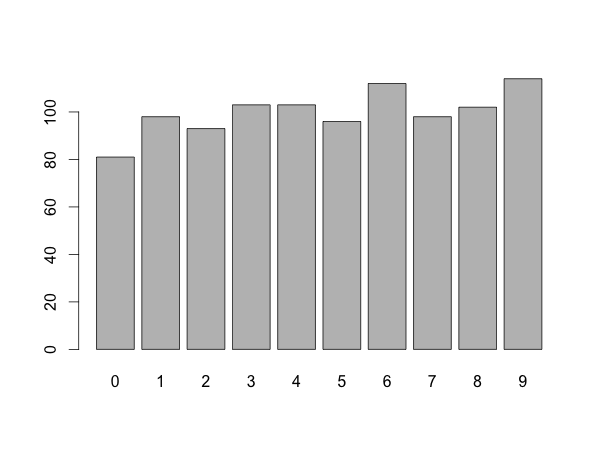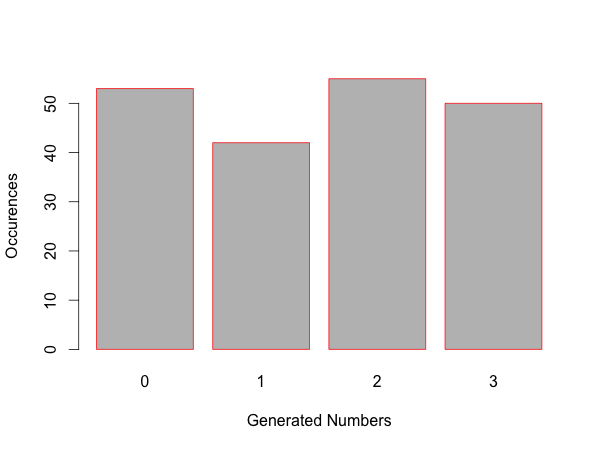```n <- floor(runif(1000)*10)
t <- table(n)
barplot(t)
```## Overview

The first line generates a list of random numbers.
The table function counts the number of occurences for each generated number. barplot creates a bar chart based on the table data.

## Getting the random numbers

runif will generate 1000 uniformly distributed numbers between 0 and 1. Because we multiply by 10 we get numbers between 0 and 10.

```runif(1000)*10
```
``` 7.346433511 5.560346758 0.762020829 2.611504081 6.582468385 9.571311316
[...]
 8.736503848 6.504455155 5.203101230 9.900331229
```

The floor function removes the digits after the decimal point, so we only get natural numbers between 0 and 9.

```floor(runif(1000)*10)
```
``` 4 4 7 5 6 4 7 6 3 3 4 5 6 3 3 8 0 3 8 4 7 0 3 9 7 4 9 7 1 2 5 6 5 3 1 5 3
[...]
 7 3 9 9 9 3 1 1 6 3 3 3 9 4 5 1 5 9 2 8 3 6 2 3 4 0 8 7 5 2 9 0 0 0 1 3 4
 3
```

## Counting value occurrences with table

```table(n)
```
```n
0   1   2   3   4   5   6   7   8   9
98  98  99 102 111 105  88 103 101  95
```

## Plotting the data with barplot

Once you have a table using barplot is really straightforward. Use the documentation if you want to specify the plot size or add axis labels.

```barplot(table(floor(runif(200)*4)), xlab="Generated Numbers", ylab="Occurrences", border="red")
```